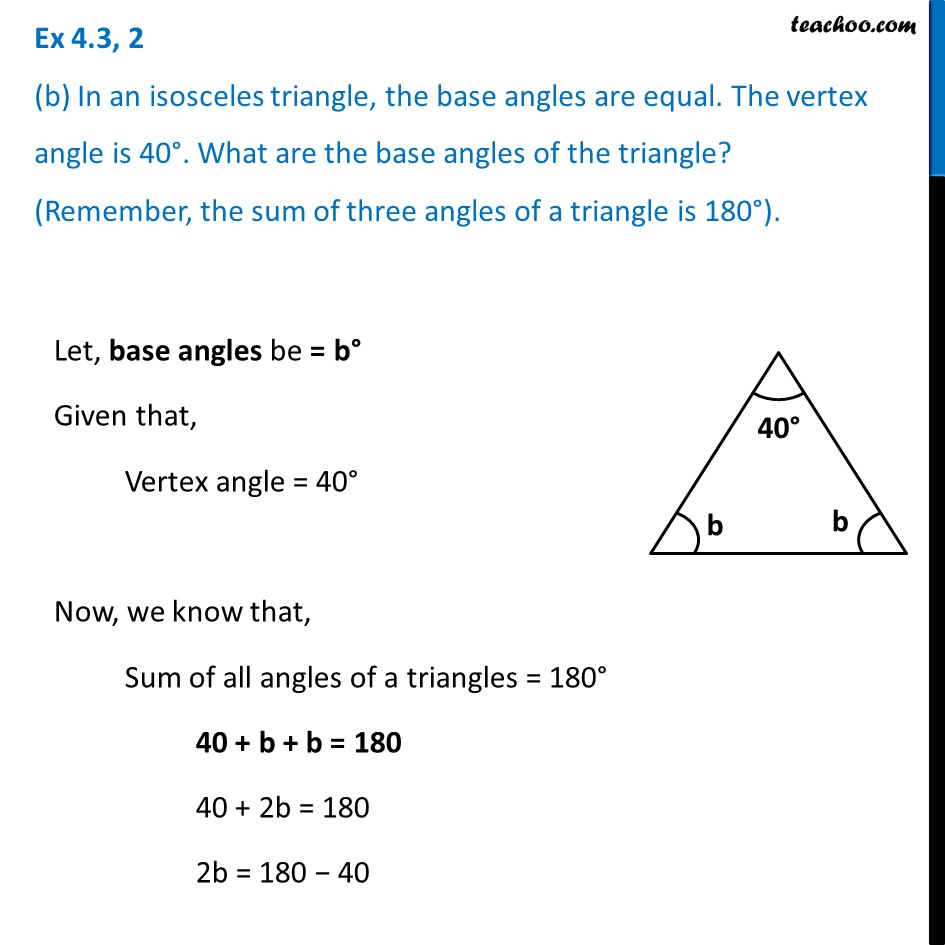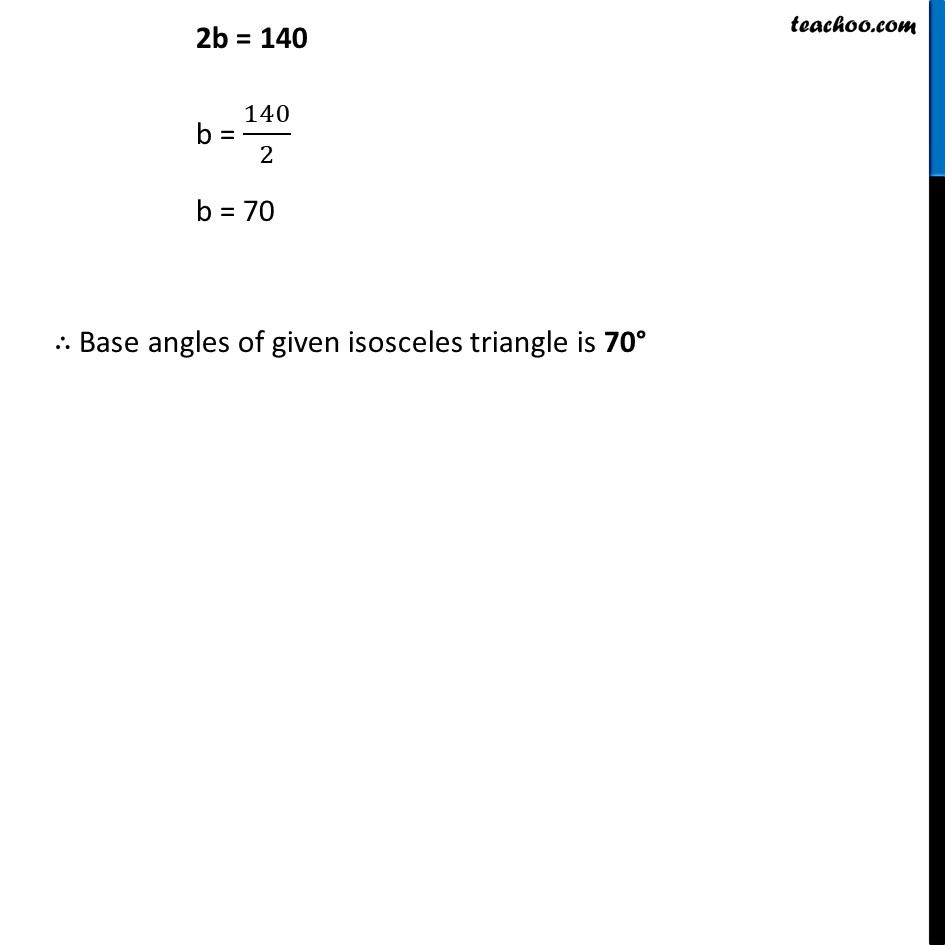Ex 4.3

Chapter 4 Class 7 Simple Equations
Serial order wiseLearn in your speed, with individual attention - Teachoo Maths 1-on-1 Class

### Transcript

Ex 4.3, 2 (b) In an isosceles triangle, the base angles are equal. The vertex angle is 40°. What are the base angles of the triangle? (Remember, the sum of three angles of a triangle is 180°).Let, base angles be = b° Given that, Vertex angle = 40° Now, we know that, Sum of all angles of a triangles = 180° 40 + b + b = 180 40 + 2b = 180 2b = 180 − 40 2b = 140 b = 140/2 b = 70 ∴ Base angles of given isosceles triangle is 70°